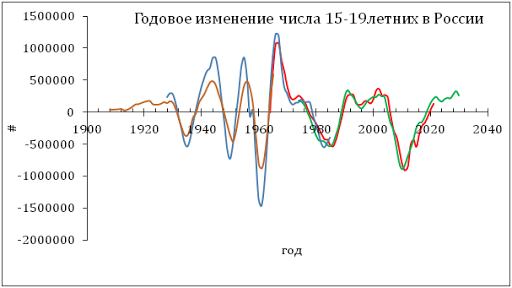## 1/21/12

### Quarterly report: the performance of share price model for Hewlett Packard

This is quarterly report of the performance of our share price model. Hewlett Packard (NYSE:HPQ) provides a good example of a successful share price prediction at a several month horizon.  We have already published our predictions at a four month horizon four times (July 2010, January 2011, March 2011, July 2011, and September 2011). All predictions were based on our concept of share pricing as decomposition into a weighted sum of two CPI components.  We calculated the evolution of the monthly closing price (adjusted for dividends and splits). Here we test and update the model using data through December 2011. The model is still accurate and robust.

Originally, the long term model for HPQ share price was defined by the index of food without beverages (FB) and that of rent of primary residency (RPR). The former CPI component led the share price by 4 months and the latter one led by 5 months. Figure 1 depicts the overall evolution of both involved indices through December 2011 (this is the reason of the time lead increase by 1 month relative to previous models where CPI were not contemporaneous). Below we present four best-fit 2-C models for HPQ(t) obtained at different times:

HPQ(t) = -3.20FB(t-4) + 2.91RPR(t-5) + 3.64(t-1990) - 50.82, July 2010
HPQ(t) = -3.34FB(t-4) + 3.41RPR(t-5) + 0.51(t-1990) - 85.44, June 2011
HPQ(t) = -3.46FB(t-4) + 3.68RPR(t-5) – 0.72(t-1990) - 99.88, September 2011
HPQ(t) = -3.40FB(t-5) + 3.60RPR(t-6) – 0.57(t-1990) – 97.72, December 2011

where HPQ(t) is the price in US dollars, t is calendar time. All coefficients have been slightly drifting but very close. This process expresses the trade-off between the linear trend in the difference between  the defining CPIs and the time trend term in the above equtions.

The predicted curves are shown in Figure 2 (March, September, and December 2011). From Figure 2, we predict the price to fall to \$20 in the first quarter of 2012 and then rise to \$25 in Q2.

Figure 1. Evolution of the price of FB and RPR.

Figure 2. Observed and predicted HPQ share prices in March, September, and December 2011. The contemporaneous prediction is shown by solid red line.  High and low prices are shown by dashed lines.